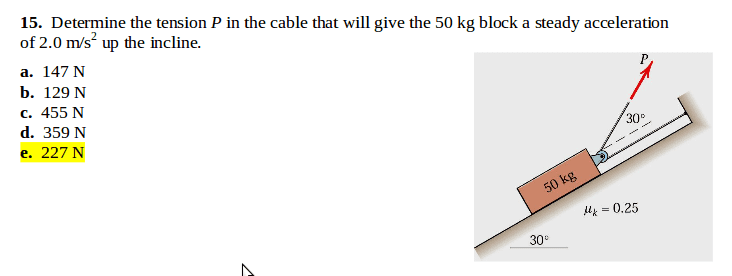# Object on Inclined plane with Force applied and Friction

Javier21

## Homework Statement

Scroll down for pic related

Determine the tension
in the cable P that will give the 50 kg block a steady accelerationof 2.0 m/s^2 up the incline.

## Homework Equations

Where am I going wrong?

## The Attempt at a Solution

Let Fa=force applied

I calculated the DeltaF to be 100N
(F=ma, F=50(2), F=100)

I calculated the force normal to be 424.8N
(Fn=50*9.81cos30)

I calculate Fg in x direction to be 245.25
(50*9.81sin30)

I calculated Fres to be (106.7-0.125Fa)
Fa is moving opposite from the fg, so I figured less force needs to be canceled out by the Fn. Will depend on force applied.
(Fn-Fasin30)(u), (426.8-0.5Fa(.25))

Since the acceleration in upwards on the plane, both the Fg(x) and Fres will be resistive forces. The Fa thus will have to overcome both of these forces added together.

Everything included:

Fa-Fres-Fg(x)+Facos(∅)=0
100-245.25- (106.7-0.125Fa)+Facos30=0

145.25+(106.7-0.125Fa)/Cos30)=Fa

And I tried subsituting the values of the multiple choice answers for Fa, but none of them worked out correctly.## Answers and Replies

Homework Helper
I calculated the force normal to be 424.8N
(Fn=50*9.81cos30)
That would be taking only the weight into account... doesn't the force P also have a normal component?

note: 30deg is a nice angle.

sin(30)=1/2 and cos(30)=sqrt(3)/2

Javier21
That would be taking only the weight into account... doesn't the force P also have a normal component?

note: 30deg is a nice angle.

sin(30)=1/2 and cos(30)=sqrt(3)/2

I accounted for that during the force resistance in a later calculaton, see:

Note that I set P=Fa

I calculated Fres to be (106.7-0.125Fa)
Fa is moving opposite from the fg, so I figured less force needs to be canceled out by the Fn. Will depend on force applied.
(Fn-Fasin30)(u), (426.8-0.5Fa(.25)

But yeah I shouldnt have stated force normal that way. And yeah I know about specail traingles, could have simplified it more though I guess. Does the thoery seem correct though? I think the angle isalso incorrect for the force applied in the normal direction.

And solving for Fa i get 289.2N

100-245.25-106.7/(-sqrt(3)/2)+0.125)=fa

Last edited:
Homework Helper
No - the theory does not seem correct.

Probably because of the piece-meal approach making it difficult to tell what you have done... for you as well as me. You appear to have been trying to take each force separately and figuring what would oppose it and what would be needed to get it to do something else. That won't work.
The theory you are supposed to be using is ƩF=ma. You need to use all the forces in the ƩF.

Take it one step at a time.

Draw a free body diagram:
Look at everything that is pulling on the block - write a force for each one. Draw the arrows on the diagram.
Then work out how to orient your coordinate system, and resolve the off-axis forces against it.
Then sum the forces.

Otherwise you will miss stuff out.

Javier21
No - the theory does not seem correct.

Probably because of the piece-meal approach making it difficult to tell what you have done... for you as well as me. You appear to have been trying to take each force separately and figuring what would oppose it and what would be needed to get it to do something else. That won't work.
The theory you are supposed to be using is ƩF=ma. You need to use all the forces in the ƩF.

Take it one step at a time.

Draw a free body diagram:
Look at everything that is pulling on the block - write a force for each one. Draw the arrows on the diagram.
Then work out how to orient your coordinate system, and resolve the off-axis forces against it.
Then sum the forces.

Otherwise you will miss stuff out.

I figured it out. I was not aware that the Force P(when using a pully) acted parrel to the plane in addition to the cosine of the force P(1+cos∅). I also made algebraic mistake in placing the delta force in x direction on the same side as the other forces. Thanks for your help never the less, got me to research the topic more.